Next: About this document ... Up: Labs and Projects for Previous: Labs and Projects for

Subsections

# Applications of exponential and logarithmic functions

## Purpose

The purpose of this lab is to use Maple to study applications of exponential and logarithmic functions. These are used to model many types of growth and decay, for example bacterial growth and radiaoctive decay. This lab also describes applications of exponential and logarithmic functions for heating and cooling and to medicine dosage

## Background

### Exponential growth

The simple model for growth is exponential growth, where it is assumed that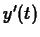is proportional to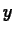. That is,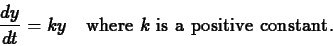Separating the variables and integrating (see section 4.4 of the text), we have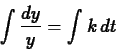so thatIn the case of exponential growth, we can drop the absolute value signs around, becausewill always be a positive quantity. Solving for, we obtain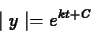which we may write in the form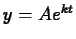, where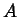is an arbitrary positive constant.

### Exponential Decay

In a sample of a radioactive material, the rate at which atoms decay is proportional to the amount of material present. That is,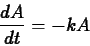where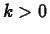is a constant. This is the same equation as in exponential growth, except thatreplaces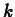. The solution is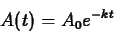where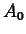is a positive constant. Physically,is the amount of material present at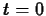.

Radioactivity is often expressed in terms of an element's half-life. For example, the half-life of carbon-14 is 5730 years. This statement means that for any given sample of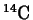, after 5730 years, half of it will have undergone decay. So, if the half-life is of an element Z is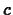years, it must be that, so that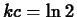and.

### Newton's law of cooling

What is usually called Newton's law of cooling is a simple model for the change in temperature of an object that is in contact with an environment at a different temperature. It says that the rate of change of the temperature of an object is proportional to the difference between the object's temperature and the temperature of the environment. Mathematically, this can be expressed as the differential equationwhereis the constant of proportionality and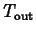is the temperature of the environment. Using a technique called separation of variables it isn't hard to derive the solution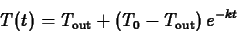whereis the temperature of the object at.

## Maple commands

The main functions you need are the natural exponential and natural logarithm. The Maple commands for these functions are exp and ln. Here are a few examples.
  >
f := x -> exp(-2*x);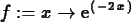>
simplify(ln(3)+ln(9));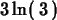>
ln(exp(x));>
simplify(ln(exp(x)),assume=real);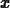The assume=real is needed in the command above, because Maple usually works with complex variables.
  >
solve(exp(-3*x)=0.5,x);>
plot(log(x),x=0..100);


Sometimes you need to use experimental data to determine the value of constants in models. For example, suppose that for a particular drug, the following data were obtained. Just after the drug is injected, the concentration is 1.5 mg/ml (milligrams per milliliter). After four hours the concentration has dropped to 0.25 mg/ml. From this data we can determine values of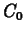andas follows. The value ofis the initial concentration, so we haveTo find the value ofwe need to solve the equationwhich we get by plugging in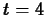and using the data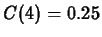. Maple commands for solving forand defining and plotting the function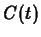are shown below.
  >
k1 := solve(0.25=1.5*exp(-4*k),k);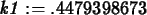>
C1 := t -> 1.5*exp(-k1*t);>
plot(C1(t),t=0..6);


## Exercises

1. In 1935 Charles F. Richter of Cal Tech developed a scale for measuring the magnitude of earthquakes. The Richter Scale formula is given by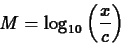where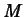is the magnitude of the earthquake,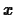is the amplitude of the largest seismic wave as measured on a standard seismograph 100 kilometers from the epicenter andis the amplitude of a reference earthquake of amplitude 1 micron ( 1 micron is 0.001 mm) on a standard seismograph at the same distance from the epicenter.
1. In 1989, the San Francisco Bay area suffered severe damage from an earthquake of magnitude 7.1. However, the damage was not nearly as extensive as that caused by the great quake of 1906, which has been estimated to have had the magnitude 8.3. What is the ratio of the amplitude of the 1906 quake to the 1989 quake? The largest earthquake magnitude ever measured was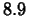for an earthquake in Japan in 1933. Determine the ratio of the amplitude of this earthquake to that of the 1906 San Francisco earthquake.

2. Worldwide use of the Internet is increasing at an exponential rate. If traffic on the Internet is doubling every 150 days, find the exponential growth rate constant.

3. Suppose that the last bit of ice in a picnic cooler has melted. How long will it take for the temperature inside to reach? Use Newton's law of cooling to model this, using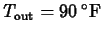,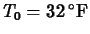and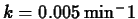.

4. The worst nuclear accident in history happened in 1986 at the Chernobyl nuclear plant near Kiev in the Ukraine. An explosion destroyed one of the plant's four reactors, releasing large amount of radioactive isotopes into the atmosphere.

Consider 10 grams of the plutonium isotope Pu-239 released in the Chernobyl nuclear accident. This isotope has a half-life of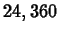years. How long will it take for the 10 grams to decay to 1 gram?Next: About this document ... Up: Labs and Projects for Previous: Labs and Projects for
Jane E Bouchard
2001-04-16